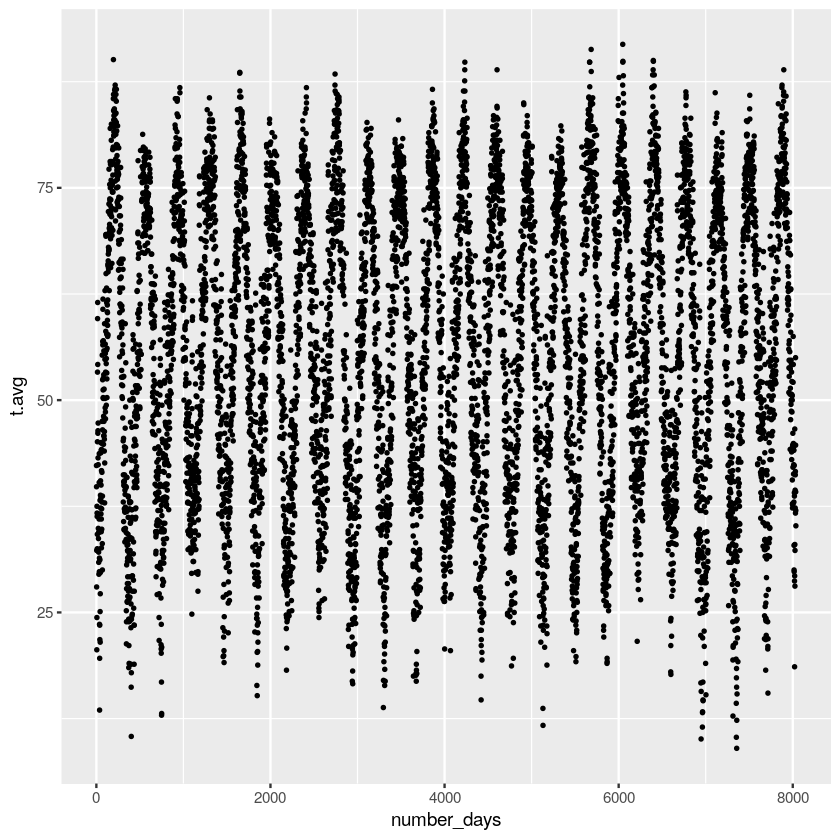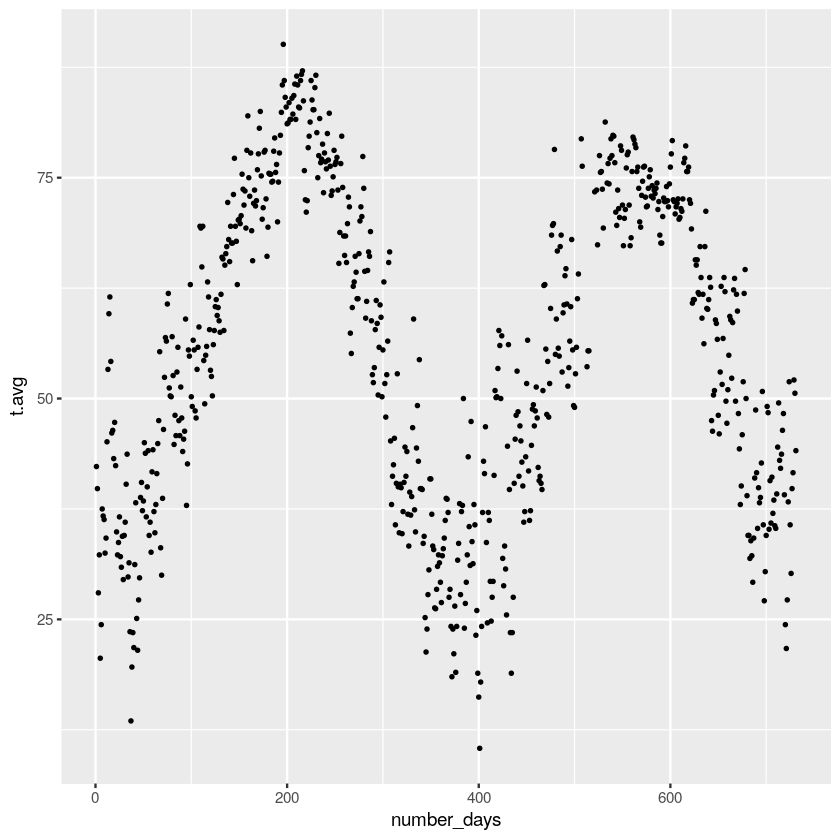# CDS-102: Lab 13 Workbook¶

## Name: Helena Gray¶

### April 26, 2017¶

In :
# Run this code block to load the Tidyverse package
.libPaths(new = "~/Rlibs")
library(tidyverse)
library(modelr)
# the model.sin function

Loading tidyverse: ggplot2
Conflicts with tidy packages ---------------------------------------------------
filter(): dplyr, stats
lag():    dplyr, stats

In :
# To change the size of any plots, copy the code snippet
# below, uncomment it, and set the size of the width
# and height.
# Note: All subsequent figures will use the same size,
# unless you change the options() snippet and run it
# again.

# options(repr.plot.width=6, repr.plot.height=4)

In :
# Test that dataset loaded
glimpse(t.data)

Observations: 8,036
Variables: 4
$month <int> 1, 1, 1, 1, 1, 1, 1, 1, 1, 1, 1, 1, 1, 1, 1, 1, 1, 1, 1, 1, 1...$ day   <int> 1, 2, 3, 4, 5, 6, 7, 8, 9, 10, 11, 12, 13, 14, 15, 16, 17, 18...
$year <int> 1995, 1995, 1995, 1995, 1995, 1995, 1995, 1995, 1995, 1995, 1...$ t.avg <dbl> 42.3, 39.8, 28.0, 32.3, 20.6, 24.4, 37.5, 36.7, 36.3, 32.5, 3...

In :
# Test that model.sin loaded
print(model.sin(T = 1, n = 0, x = 1/3))

 0.8660254


In :
ndays <- nrow(t.data)
t.data.filtered <- filter(t.data, t.avg > -99)


In :
ggplot(t.data.filtered) + geom_point(aes(x=number_days, y=t.avg), size=0.5)In :
 t.data.y9596<-filter(t.data.filtered, c(year==1995|year==1996))

ggplot(t.data.y9596) + geom_point(aes(x=number_days, y=t.avg), size=0.5)In the northern hemisphere we tend to be hottest in July and coldest in January. The difference between January 1st and July 1st is roughly 180 days. The time it takes the Earth to make one rotation around the sun is roughly 365 days or a year.

In :
model.T<-365

In :
mod_n_0 <- lm(t.avg~model.sin(T=model.T, n=0, x=number_days),
data=t.data.filtered)

mod_n_1 <- lm(t.avg~model.sin(T=model.T, n=1, x=number_days),
data=t.data.filtered)

mod_n_2 <- lm(t.avg~model.sin(T=model.T, n=2, x=number_days),
data=t.data.filtered)

mod_n_3 <- lm(t.avg~model.sin(T=model.T, n=3, x=number_days),
data=t.data.filtered)

mod_n_4 <- lm(t.avg~model.sin(T=model.T, n=4, x=number_days),
data=t.data.filtered)

mod_n_5 <- lm(t.avg~model.sin(T=model.T, n=5, x=number_days),
data=t.data.filtered)

mod_n_5
mod_n_4
mod_n_3
mod_n_2
mod_n_1
mod_n_0

Call:
lm(formula = t.avg ~ model.sin(T = model.T, n = 5, x = number_days),
data = t.data.filtered)

Coefficients:
(Intercept)
56.16
model.sin(T = model.T, n = 5, x = number_days)
17.23

Call:
lm(formula = t.avg ~ model.sin(T = model.T, n = 4, x = number_days),
data = t.data.filtered)

Coefficients:
(Intercept)
56.18
model.sin(T = model.T, n = 4, x = number_days)
21.57

Call:
lm(formula = t.avg ~ model.sin(T = model.T, n = 3, x = number_days),
data = t.data.filtered)

Coefficients:
(Intercept)
56.19
model.sin(T = model.T, n = 3, x = number_days)
20.14

Call:
lm(formula = t.avg ~ model.sin(T = model.T, n = 2, x = number_days),
data = t.data.filtered)

Coefficients:
(Intercept)
56.18
model.sin(T = model.T, n = 2, x = number_days)
13.29

Call:
lm(formula = t.avg ~ model.sin(T = model.T, n = 1, x = number_days),
data = t.data.filtered)

Coefficients:
(Intercept)
56.154
model.sin(T = model.T, n = 1, x = number_days)
2.862

Call:
lm(formula = t.avg ~ model.sin(T = model.T, n = 0, x = number_days),
data = t.data.filtered)

Coefficients:
(Intercept)
56.143
model.sin(T = model.T, n = 0, x = number_days)
-8.308


In :
grid <- data_grid(data=t.data.y9596,
number_days=seq_range(number_days, n=1000,
expand=0.05))
grid <- gather_predictions(grid, mod_n_0, mod_n_1, mod_n_2,
mod_n_3, mod_n_4,
mod_n_5, .pred="t.avg")

In :
ggplot(t.data.y9596) +
geom_point(aes(number_days, t.avg)) +
geom_line(data=grid, aes(number_days, t.avg),
color="red", size=1) +
facet_wrap(~model)